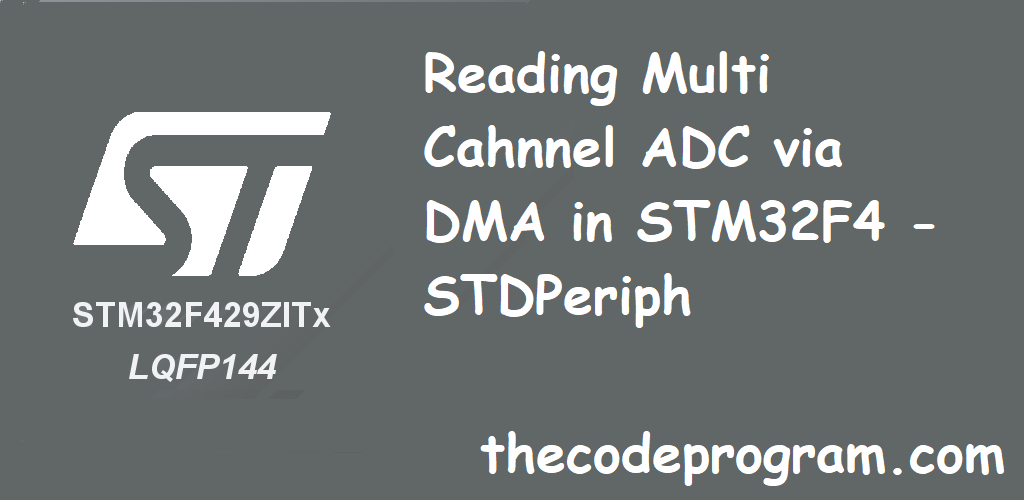Hello everyone, in this article I will explain how can we read multi channel ADC via DMA in STM32F4 ARM based micropocessor. Iwill use STM32F429ZI Nucleo board. I will built the program in uVision Keil and Std Periph drivers.

Firstly, I have to say that If you have no idea how to configure the DMA then you have to check the my article which I posted How can we configure the DMA and ADC from this link.

Now we can start to code our program.

First we need to create our Initialization Structures. We need GPIO, DMA and ADC init type definitions. You can find them below code block. After definition of these periphals we have to enable rrelated buses to activate and use these periphal drivers.
``````
DMA_InitTypeDef       DMA_InitStruct;
GPIO_InitTypeDef      GPIO_InitStruct;

//Enabling the related buses
RCC_AHB1PeriphClockCmd( RCC_AHB1Periph_GPIOA | RCC_AHB1Periph_DMA2 , ENABLE);
``````

From now we will first configure GPIO to use required pins. And then we will configure the DMA and ADC.
Below code block you can find the GPIO configurations.

``````
GPIO_InitStruct.GPIO_Pin = GPIO_Pin_2 | GPIO_Pin_3 | GPIO_Pin_4 | GPIO_Pin_5 | GPIO_Pin_6 | GPIO_Pin_7;
GPIO_InitStruct.GPIO_Mode = GPIO_Mode_AN;
GPIO_InitStruct.GPIO_PuPd = GPIO_PuPd_NOPULL ;
//Initialize the GPIOA with above configurations
GPIO_Init(GPIOA, &GPIO_InitStruct);
``````

We will keep the channels' analog voltage values in the related variable. Below code block we will define a variable named ADCValues with 6 elements. We will set this variable as target memory variable.

``````
``````

Now below code block we will configure the DMA.
We are going to use ADC2 to read voltage values. ADC2 connected to DMA2 Channel1 Stream2. So we will enable the DMA2 and set the mode as PeriphalToMemory. We will transfer data from periphal to the memory.

``````
//We will set the channel as DMA_Channel_1
DMA_InitStruct.DMA_Channel = DMA_Channel_1;
//Set the direction as Periphal to Memory
DMA_InitStruct.DMA_DIR = DMA_DIR_PeripheralToMemory;
//We will use 6 adc channel. and buffer size is 6
DMA_InitStruct.DMA_BufferSize = 6;
DMA_InitStruct.DMA_PeripheralInc = DMA_PeripheralInc_Disable;
DMA_InitStruct.DMA_MemoryInc = DMA_MemoryInc_Enable;
//We will set data sizes as 16-bit
DMA_InitStruct.DMA_PeripheralDataSize = DMA_PeripheralDataSize_HalfWord;
DMA_InitStruct.DMA_MemoryDataSize = DMA_MemoryDataSize_HalfWord;
//DMA circular mode enables the dma to start when it is done
DMA_InitStruct.DMA_Mode = DMA_Mode_Circular;
DMA_InitStruct.DMA_Priority = DMA_Priority_High;
DMA_InitStruct.DMA_FIFOMode = DMA_FIFOMode_Disable;
DMA_InitStruct.DMA_FIFOThreshold = DMA_FIFOThreshold_HalfFull;
DMA_InitStruct.DMA_MemoryBurst = DMA_MemoryBurst_Single;
DMA_InitStruct.DMA_PeripheralBurst = DMA_PeripheralBurst_Single;
//Initialize the DMA
DMA_Init(DMA2_Stream2, &DMA_InitStruct);
//Enable the DMA
DMA_Cmd(DMA2_Stream2, ENABLE);
``````
``````

//Enable the continuos adc voltage sampling
//How many analog voltage values we will read
//Initialize the ADC with above configurations.
``````
After initialization of the ADC and DMA we need to specify the ADC channels to use. I specified the GPIO pins and ADC channels. Below list has been taken from STM32F4 datasheet.
Now we have to set the order to read them. Below code block will order them.
``````
``````
After it now will are going to initialze the ADC
``````
// Enable DMA request after last transfer
``````

As I mentioned the on this article DMA transfers the data into variable but you probably can not see the changings in the debug window. You can see the changing with a led blanking application. Below code block is an example of this. PB14 pin connected to LD3 red led on the STM32F429ZI Nucleo.

``````
RCC_AHB1PeriphClockCmd( RCC_AHB1Periph_GPIOB, ENABLE);
GPIO_InitStructure.GPIO_Mode = GPIO_Mode_OUT;
GPIO_InitStructure.GPIO_Pin = GPIO_Pin_14;
GPIO_InitStructure.GPIO_Speed = GPIO_Speed_50MHz;
GPIO_InitStructure.GPIO_OType = GPIO_OType_PP;
GPIO_InitStructure.GPIO_PuPd = GPIO_PuPd_NOPULL;
//Activate the GPIO configurations
GPIO_Init(GPIOB, &GPIO_InitStructure);

while(1)
{
GPIO_SetBits(GPIOB, GPIO_Pin_14);
GPIO_ResetBits(GPIOB, GPIO_Pin_14);
}
``````

You do not need any while loop and NVIC IRQ handler to read analog voltage value. Implementation is enough to read analog voltages.

Have a good Multi Channel Analog voltage reading.

Burak hamdi TUFAN

12/04/2020

23/05/2020

29/05/2020